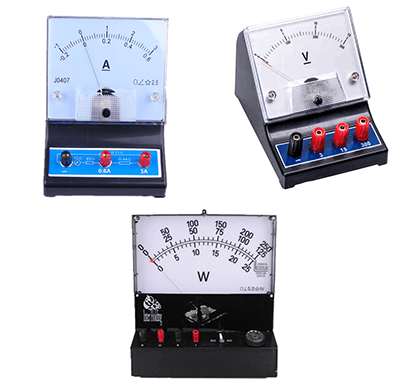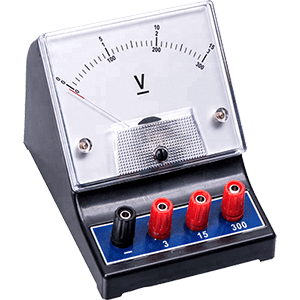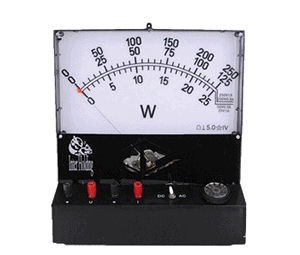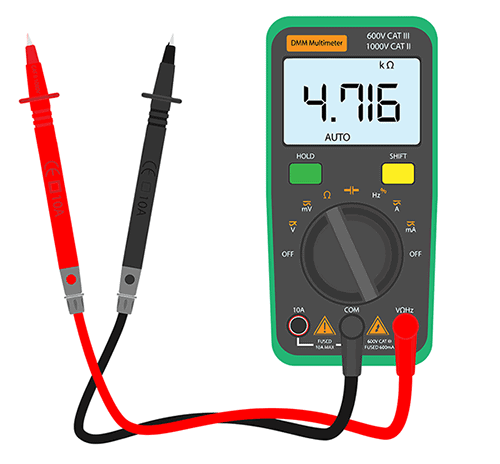# Types of Electrical and Electronic Instruments

In Electronics, there are different types of electrical and electronic instruments that can indicate or records some physical values that are said to be called meters.

A Meter is an instrument or a device that measures voltage, current, resistance, power, capacitance, temperature, frequency, etc. Many types of electronic instruments measure these electrical quantities accurately. Electronic Industries and Research labs use different measuring instruments to design, diagnose errors and faults in the electrical system, and also to rectify it.

Measuring these quantities is important to determine the various aspects of the system. For example, to measure the potential difference across the element, we use a voltmeter and the amount of current flowing in the circuit ammeter measures the current.Different analog meter instruments

The instruments which are used to indicate the value of electrical quantity when it is measured are called indicating instruments. These instruments have a pointer which moves over a scale when an electrical quantity being measured is passed through a particular meter.

## Analog Instruments

The proper operation of electrical or electronic instruments (analog) depends on the following torques:

• Deflecting torque
• Controlling torque
• Damping torque

### Deflecting torque

The deflecting torque makes the pointer move away from the zero position to the desired reading. It utilizes the physical effects of electric current or voltage to produce a mechanical force which causes the deflection of pointer.

### Controlling torque

Controlling torque limits the movement of the pointer. This torque ensures that the pointer does not go beyond the range of scale by opposing the deflecting torque. The pointer comes to zero when the deflecting and controlling torques are equal to each other.

There are two methods for controlling torque:

1.  Spring control method
2. Gravity control method

#### Spring control method

In this method, there are two phosphor bronze springs are used and are attached to the shaft or spindle. Springs should be made of non-magnetic material of low specific resistance. They are wounded in the opposite direction to compensate against temperature changes. The pointer is also attached to the shaft.

The controlling torque is depending on the twisting of springs. When the moving system deflected, spring twists in opposite direction. This results in producing a restoring torque which is proportional to the angle of deflection of pointer.

Hence, the pointer stops and comes to zero position when the deflection and controlling torque are equal (Td=Tc).

Advantages:

• The scale is uniform
• It can be placed in any position
• The weight of the instrument is not heavier in as the springs are light weighted

Disadvantages:

• The accuracy will be lost if the springs get deteriorated
• Variations in temperature can affect the spring

#### Gravity Control Method

Here weights are used to balance the movement of pointe. A small adjustable weight is attached to the spindle of the moving system. Whenever a pointer tends to move from its original position, it produces a controlling torque due to gravitational pull.

Another weight can also be added for balancing and zero adjustments and this is called balanced weight.

Advantages:

• Cheap
• The temperature can’t be affected
• It doesn’t deteriorate

Disadvantages:

• Scale is non-linear
• It will increase the weight of the instrument
• The instrument needs to be in a vertical position

### Damping torque

Damping torque makes the pointer to return to zero position gradually after the measurement. It minimizes the oscillations of the pointer. If there is no damping torque, then the pointer will keep on moving to its final deflected position for some time before coming to rest, due to the inertia of the moving system.

Thus, a force which is required to make the pointer comes to its original position is called damping force.

The damping torque can be produced by the following methods:

1. Air-friction damping
2. Eddy current damping

#### Air-Friction Damping

There are two methods employed using air-friction damping.

1. In one method – It has an air chamber and a light piston made of aluminium which is attached to the moving system.

The piston is placed inside the air chamber which moves inside the chamber along with the pointer and it is closed at one end. When the piston moves inside the chamber, the air inside the closed space is compressed and the pressure of air is developed which opposes the motion of the piston. The change in the air pressure when the piston moves provide a necessary damping force.

This method is used in moving iron meter and it is simple and cheap. Care must be taken while using this kind of instrument that the piston is not bent and it should not touch the walls of the chamber because it will cause a serious error in the deflection while reading.

1. In the other method, a light vane which is made up of aluminum attached to the spindle where a pointer is also attached. It moves in a quadrant shaped air chamber. When the pointer moves, the vane also moves and compresses the air. The pressure of the compressed air provides the necessary damping force to reduce the oscillations of the pointer.

The vane displaces air and a damping force is created on the vane that produces a damping torque on the spindle. When the spindle is at rest, the damping force is zero and when the movement is quicker the damping force is greater.

The vane should not touch the chamber walls if it happened it will cause serious errors in the deflection of pointer.

#### Eddy Current Damping

Eddy currents are induced in the conductor due to the change in the magnetic field produced by the permanent magnet. This damping is used in permanent magnet moving coil instruments.

There are two methods for producing eddy current damping:

1. This method employs a permanent magnet and a coil. The coil is wounded on a metal former made of aluminium in which the eddy current is produced when the coil moves in the permanent magnetic field.
1. This method employs a conducting disc (non-magnetic material) made up of aluminum attached to the spindle and the pointer. When the spindle moves, the disc also rotates in the magnetic field. The disc cuts the magnetic lines of force of the permanent magnet and induces eddy current in it. The induced eddy current opposes the movement and a damping force is developed.

## Types of Electrical and Electronic Instruments

There are different types of electronic measuring Instruments or meters for measuring electrical quantities. They are:

• Voltmeter
• Ammeter
• Wattmeter
• Ohmmeter
• Multimeter
• Q-Meter

### VoltmeterAnalog Voltmeter

The Voltmeter measures the potential difference between the two elements in a circuit. The connection between the elements is in parallel because it has high resistance.

The parallel connection of voltmeter with the circuit element, the amount of current through the voltmeter is very less. This stops the current through the circuit.  Otherwise, it will not give a proper reading. The units of Voltage is   Volts (V).

There are two types of Voltmeter: Analog voltmeter and Digital voltmeter.

The Analog meter induces torque by applying the voltage and the pointer moves over a scale.  It works on the principle of moving iron or moving coil meters. A digital voltmeter has a display that gives a numerical result.

### AmmeterAnalog Ammeter

An Ammeter measures the current flowing in the circuit. The unit Electric current is Ampere (A).

An ammeter is a low impedance device. The connection is in series and current is same in a series circuit (measurand current passes through the circuit) and there will be a voltage drop across it because of low resistance. So that the meter can give an accurate reading.

If the circuit is in parallel connection mode, it can cause a short circuit. It works based on the principle of moving iron or moving coil meters.

### WattmeterWattmeter

A Watt measures the power flowing in a circuit. It does a complex job by measuring the voltage and current in a circuit and multiplies it to give the value of power. The unit of power is watts. The wattmeter works based on the principle of electrodynamic and moving coil meter.

### Ohmmeter or Megger

Ohmmeters measure the resistance of an element in a circuit. The unit of resistance is the ohm. There are different meters are available: Milli ohmmeter, Micro ohmmeter, Mega ohmmeter.

Milli ohmmeter measures low resistance, Whereas Micro ohmmeter measures extremely low resistance, and Mega ohmmeter measure high resistance. There are two types of ohmmeters available: Series ohmmeter and shunt ohmmeter. They work based on the principle of moving coil meter.

### MultimeterDigital Multimeter

A Multimeter is a multi-function measurement device. It can measure voltage, current, and resistance. It is also known as VOM (volt-ohm-milliammeter). There are two types of multimeters available: Analog multimeter and Digital multimeter.  An Analog Multimeter is based on the principle of moving coil meter.

To read the numerical measurements, Digital Multimeter has a digital display on which we can read different values. The rotary switch points to particular electrical quantities and shows the corresponding readings with units. Nowadays, a digital multimeter is widely used because of its efficiency and faster reading.

### LCR Meter or Q-Meter

The LCR meter (Q-Meter) measures the Inductance, Capacitance, and resistance in a circuit or device. It also measures the phase angle when measuring the voltage and current through the ‘Device Under Test’ (DUT). Finally, the impedance calculation is taken from the phase angle.

There are two types of LCR meters. They are Hand-held and Benchtop.  Handheld LCR meter is easy to carry and maintain but less accurate. Whereas Benchtop meters are highly accurate with additional features such as AC calibration of resistance, inductance, and capacitance. To test sensors and components on PCB, an LCR meter is useful.

## What is the Loading Effect?

The electrical measuring instruments draw power from the measurement circuit. This power enables the circuit to operate in the correct manner. Depending on the power consumption, the circuit condition may change and the loading effect takes place. In AC circuits, the instrument impedance changes with the frequency and changes the loading effect.

This problem occurs in the voltmeter and shows error readings. To eliminate or reduce the loading effect, use a voltmeter with higher sensitivity.

## Summary

Electronic Measuring Instruments measures parameters like voltage, current, resistance, wattage, etc. They use moving coil and moving iron mechanisms as their working principle. Different torques are responsible for the operation of the instrument.

The different types of electrical and electronic instruments are a voltmeter, ammeter, wattmeter, ohmmeter, LCR meter, and a multimeter. The meter has a pointer which moves over a scale whenever the quantity passes through the meter.